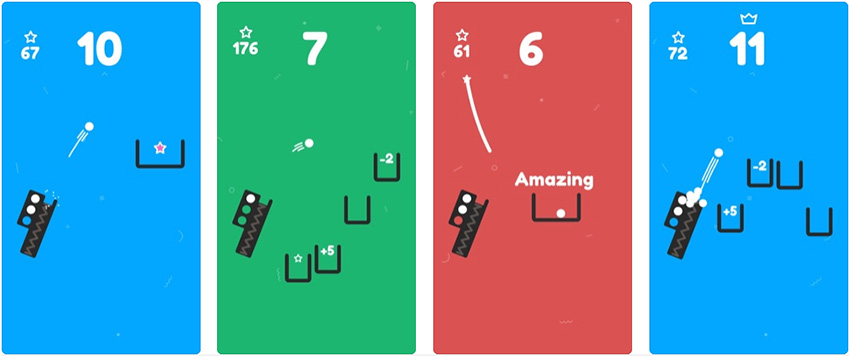# Build a HTML5 game like “Pocket Snap” using Phaser and Matter physics

Yes, Ketchapp did it again. Their lastest game, Pocket Snap, is another quick and fun hyper casual game.

Just tap, hold and snap the ball into the box

You can get it both for iOS and Android.Although there is not reason to use a complex physics engine to create a prototype of the game, and I will show you how to do it in another post, I wanted to create the engine entirely using Matter physics, just to practice with constraints. And trigonometry!

Have a look at the result:

Tap and hold to charge, release to fire the ball. The game restart a couple of seconds after you fired the ball to let you see how many random customization are possible.

The core of the script, apart from some heavy trigonometry to properly place and rotate the cannon, is the use of constraints.

We start with a constraint with full stiffness whose length is manually reduced just to lower the stiffness and raise the length when it’s time to fire the ball.

Being a physics only simulation, you also have to play with friction and keep in mind Matter physics does not feature continue collision detection – this post about Matter and CCD will explain you everything – so you will get strange results if too much force is applied to the trigger.

Look at the commented source code:

```let game;
let gameConfig = {
type: Phaser.AUTO,
scale: {
mode: Phaser.Scale.FIT,
autoCenter: Phaser.Scale.CENTER_BOTH,
parent: "thegame",
width: 600,
height: 600
},
scene: playGame,
physics: {
default: "matter",
matter: {
gravity: {
y: 1
},
debug: true,
debugBodyColor: 0xff00ff,
debugWireframes: false
}
}
}
game = new Phaser.Game(gameConfig);
window.focus();
}
class playGame extends Phaser.Scene{
constructor(){
super("PlayGame");
}
create(){

// matter settings
this.matter.world.update30Hz();
this.matter.world.setBounds(10, 10, game.config.width - 20, game.config.height - 20);

// random cannon properties
let angle = Phaser.Math.Between(45, 135);
let width = Phaser.Math.Between(20, 50);
let length = Phaser.Math.Between(120, 250);
let tickness = Phaser.Math.Between(10, 20);
let position = new Phaser.Math.Vector2(game.config.width / 2, 550);

// bottom body
let bottomWidth = width + tickness * 2;
let bottomBody = this.matter.add.rectangle(position.x, position.y, bottomWidth, tickness, this.setProperties(true, angle));

// some trigonometry useful to find the origins of cannon side bodies
let bottomCathetus = (width + tickness) / 2;
let sideCathetus = (length + tickness) / 2;
let hypotenuse = Math.sqrt(Math.pow(bottomCathetus, 2) + Math.pow(sideCathetus, 2));
let bottomAngle = Phaser.Math.RadToDeg(Math.asin(sideCathetus / hypotenuse));

// side body 1
let firstSideOrigin = this.moveBy(position, hypotenuse, 90 - bottomAngle - angle)
this.matter.add.rectangle(firstSideOrigin.x, firstSideOrigin.y, tickness, length, this.setProperties(true, angle));

// side body 2
let secondSideOrigin = this.moveBy(position, hypotenuse, bottomAngle - 90 - angle)
this.matter.add.rectangle(secondSideOrigin.x, secondSideOrigin.y, tickness, length, this.setProperties(true, angle));

// trigger
let triggerOrigin = this.moveBy(position, (length - (width * 3 - tickness) / 2), -angle)
let trigger = this.matter.add.rectangle(triggerOrigin.x, triggerOrigin.y, width, width, this.setProperties(false, angle));

// cannon ball
let ballOrigin = this.moveBy(position, (width + length - (width * 3 - tickness) / 2), -angle)
this.matter.add.circle(ballOrigin.x, ballOrigin.y, width / 2, this.setProperties(false, angle));

// constraint
let constraintLength = length + (tickness - width * 3) / 2;
this.constraint = this.matter.add.constraint(bottomBody, trigger, constraintLength, 1);
this.constraintFireLength = constraintLength + width;
this.constrainMinLength = width / 2 + tickness / 2;

// listeners and flags
this.input.on("pointerdown", this.charge, this);
this.input.on("pointerup", this.fire, this);
this.charging = false
}

// charge
charge(){
this.charging = true;
}

// fire: look how stiffness changes, then restart the game
fire(){
this.charging = false;
this.constraint.stiffness = 0.02
this.constraint.length = this.constraintFireLength;
delay: 3000,
callbackScope: this,
callback: function(){
this.scene.start("PlayGame");
},
});
}

// we reduce constraint length if charging
update(){
if(this.charging &amp;&amp; this.constraint.length > this.constrainMinLength){
this.constraint.length -= 1;
}
}

// utility method to create an object with body properties
setProperties(isStatic, angle){
return {
isStatic: isStatic,
friction: 0
}
}

// utility method to move a point by "distance" pixels in "degrees" direction
moveBy(point, distance, degrees){
return new Phaser.Math.Vector2(point.x + distance * Math.cos(radians), point.y + distance * Math.sin(radians));
}
};
```

I am quite happy I managed to create some kind of firing machine only using Matter, pushing the thing a little more beyond than “apply this force to this ball”.

214 GAME PROTOTYPES EXPLAINED WITH SOURCE CODE
// 1+2=3
// 10000000
// 2 Cars
// 2048
// Avoider
// Ballz
// Block it
// Blockage
// Bloons
// Boids
// Bombuzal
// Breakout
// Bricks
// Columns
// CubesOut
// Dots
// DROP'd
// Dudeski
// Eskiv
// Filler
// Fling
// Globe
// HookPod
// Hundreds
// InkTd
// Iromeku
// Lumines
// Magick
// MagOrMin
// Maze
// Memdot
// Nano War
// Nodes
// o:anquan
// Ononmin
// Pacco
// Phyballs
// Platform
// Poker
// Pool
// Poux
// Pudi
// qomp
// Racing
// Renju
// SameGame
// Security
// Sling
// Slingy
// Sokoban
// Splitter
// Sproing
// Stack
// Stringy
// Sudoku
// Tetris
// Threes
// Toony
// Turn
// TwinSpin
// vvvvvv
// Wordle
// Worms
// Yanga
// Zhed
// zNumbers